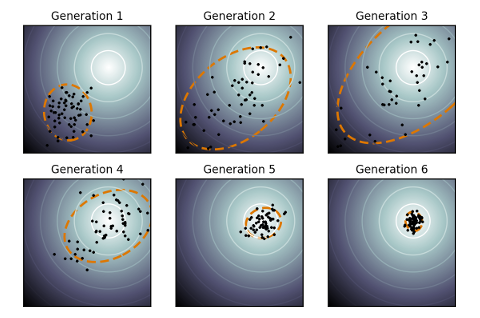# Covariance Matrix Adaptation Evolution Strategy (CMA-ES) in PythonAccording to OpenAI, Evolution Strategies are a scalable alternative to Reinforcement Learning. Where Reinforcement Learning is a guess and check on the actions, Evolution Strategies are a guess and check on the model parameters themselves. A “population” of “mutations” to seed parameters is created, and all mutated parameters are checked for fitness, and the seed adjusted towards the mean of the fittest mutations. CMA-ES is a particular evolution strategy where the covariance matrix is adapted, to cast a wider net for the mutations, in an attempt to search for the solution.

To demonstrate, here is a toy problem. Consider a shifted Schaffer function with a global minimum (solution) at f(x=10,y=10):$f(x,y)=0.5+\frac{sin^{2}[(x-10)^{2}-(y-10)^{2}]-0.5}{[1+0.001[(x-10)^{2}+(y-10)^{2}]]^{2}},\ \ \ \ \ f(10,10)=0$

The fitness function F() for CMA-ES can be treated as the negative square error between the solution being tested, and the actual solution, against the Schaffer function:$F(s_1,s_2) = -(f(s_1,s_2) - f(10,10))^2$

Therefore, the task for CMA-ES is to find the solution$(s_1=10, s_2=10)$. Given the right population size$\lambda$ and the right$\mu$ for CMA-ES, it eventually converges to a solution. With$\lambda=64$ and$\mu=0.25$, a visualization of CMA-ES as it evolves a population over generations can be seen below.

The animation below depicts how CMA-ES creates populations of parameters that are tested against the fitness function. The blue dot represents the solution. The red dots the entire population being tested. And the green dot the mean of the population as it evolves, which eventually fits the solution. You see the “net” the algorithm casts (the covariance matrix) from which the population is sampled, is adapted as it is further or closer to the solution based on the fitness score.